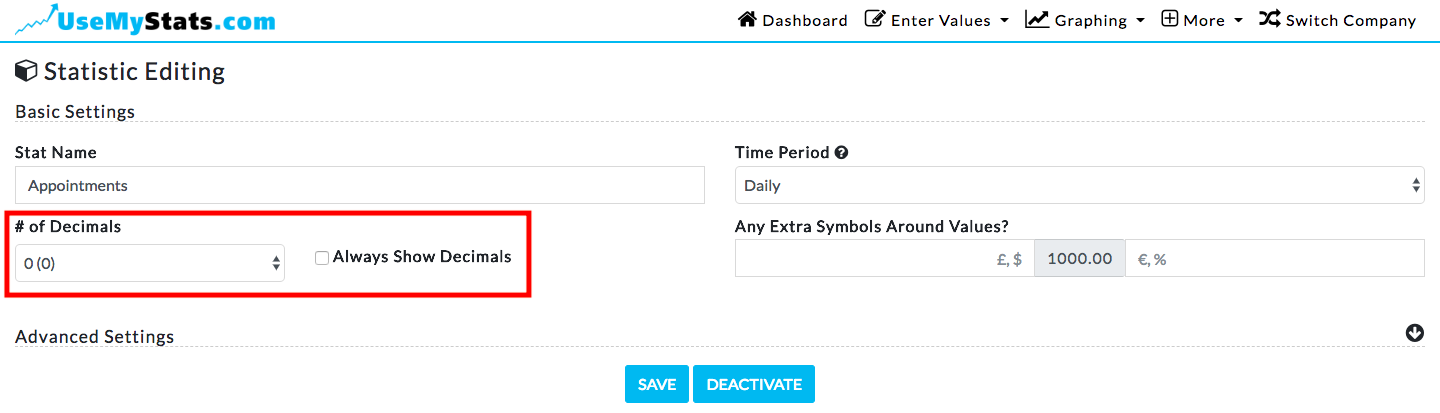On occasion, you may stumble across a graph where the intervals on the left-hand side are not always consistent, in a pattern where sometimes the interval is one larger than the last and sometimes one less. Here's an example:On the graph above, counting from the bottom, the graph goes from 62 to 60 - an interval of 2 - then from 60 to 58 - another interval of 2 - and then from 56 to 53 - an interval of 3. Why is this?

First let me assure you that the intervals are actually exactly and equally spaced, it's just the numbers that are (in this case) not exact. Again, why?

This statistic has been marked to only show whole numbers, decimals are not allowed.Now, changing the statistic to show two decimal places:And returning to the graph, you can see the intervals are actually precisely and evenly spaced at 2.15 between each line.Now, because this stat was marked as not allowed to show decimals, each number is rounded to the nearest whole number. The first number (62.00) show as 62. The next number (59.85) shows as 60.  The third (57.70) shows as 58 and the fourth (55.55) shows as 56. Each of these whole numbers have a full interval of 2 between them, since we are not taking decimals into account. The next number though, (53.40) doesn't round up to the next higher number as the three before do, it rounds down to 53. This gives the appearance that the interval between these lines is different - at 3 - but, just like the lines before, it's actually separated at a specific interval of 2.15.